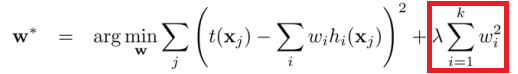# Simple L2 regularization?

Hi, does simple L2 / L1 regularization exist in pyTorch? I did not see anything like that in the losses.

I guess the way we could do it is simply have the data_loss + reg_loss computed, (I guess using nn.MSEloss for the L2), but is an explicit way we can use it without doing it this way?

Thanks

5 Likes

Hi,

The L2 regularization on the parameters of the model is already included in most optimizers, including optim.SGD and can be controlled with the weight_decay parameter as can be seen in the SGD documentation.

L1 regularization is not included by default in the optimizers, but could be added by including an extra loss nn.L1Loss in the weights of the model.

l1_crit = nn.L1Loss(size_average=False)
reg_loss = 0
for param in model.parameters():
reg_loss += l1_crit(param)

factor = 0.0005
loss += factor * reg_loss


Note that this might not be the best way of enforcing sparsity on the model though.

46 Likes

Thanks @fmassa - although I must say that’s odd that a regularization loss in included in the optimizer here. 0_0

5 Likes

Yeah, that’s been added there as an optimization, as L2 regularization is often used.

1 Like

Got it thanks!what do you recommend which would be a better way to enforce sparsity instead of L1?

1 Like

This comment might be helpful https://github.com/torch/optim/pull/41#issuecomment-73935805

Thanks for the note@fmassa does this still work? It seems that nn.L1Loss requires a target - giving the error TypeError: forward() missing 1 required positional argument: 'target'

example:

xx = nn.Parameter(torch.from_numpy(np.ones((3,3))))
l1_crit = nn.L1Loss()
l1_crit(xx)


I forgot to add the target, which in some cases would be a zero-tensor.
So something like

xx = nn.Parameter(torch.from_numpy(np.ones((3,3))))
target = Variable(torch.from_numpy(np.zeros((3,3))))
l1_crit = nn.L1Loss()
l1_crit(xx, target)

1 Like

I have two questions about L1 regularization:

• How do we backpropagate for L1 regularization? I wonder it because the term is not differentiable.

• Where can I see the implementation of L1 regularization? In the following link, there is only pass.

2 Likes

you can see the implementation of L1Loss here: https://github.com/pytorch/pytorch/blob/ecd51f8510bb1c593b0613f3dc7caf31dc29e16b/torch/lib/THNN/generic/L1Cost.c

1 Like

@fmassa, so because of PyTorch’s autograd functionality, we do not need to worry about L1 regularization during the backward pass, i.e. applying the derivative of the L1 regularization term to the gradient of the output? That will be handled by the autograd variables?

If you add the L1 regularization to the loss as I explained in a previous post, the gradients will be handled by autograd automatically.

I like to use l1_loss=F.l1_loss(xx, target=torch.zeros_like(xx), size_average=False)

2 Likes

if I want to use a custom Regularizer R can is the following code good:

batch_loss = loss(input=y_pred,target=batch_ys)
batch_loss += lambda*R


?

@fmassa You say “this might not be the best way of enforcing sparsity on the model”, and “This comment might be helpful https://github.com/torch/optim/pull/41#issuecomment-73935805 1.2k”, which comment explains to explicitly set to 0 any weights changing sign. Does this mean that you feel that L1 with explicit zeroing of weights crossing zero is an appropriate way of encouraging sparsity? Or do you mean, there are some other approach(es) that can work well?

Hi @hughperkins,

If you are interested in inducing sparsity, you might want to checkout this project from Intel AI Labs. The documentation tries to shed some light on recent research related to sparsity inducing methods.

The project includes a stand-alone Jupyter notebook that attempts to show how L1 regularization can be used to induce sparsity (by “stand-alone” I mean that the notebook does not import any code from Distiller, so you can just try it out).

Cheers,
Neta

4 Likes

Sorry for question here.
It is said that when regularization L2, it should only for weight parameters, but not bias parameters.(Is it right?)
But the L2 regularization included in most optimizers in PyTorch, is for all of the parameters in the model (weight and bias).I mean the parameters in the red box should be weight parameters only. (If what I heard of is right.)
How can I deal with it?

weight_p, bias_p = [],[]
for name, p in model.named_parameters():
if 'bias' in name:
bias_p += [p]
else:
weight_p += [p]

optim.SGD(
[
{'params': weight_p, 'weight_decay':1e -5},
{'params': bias_p, 'weight_decay':0}
],
lr=1e-2, momentum=0.9
)


Code here can deal with the problem above, is it right?1 Like

We do regularization to handle the high variance problem (overfitting). It’s good if the regularization includes all the learnable parameters (both weight and bias). But since bias is only a single parameter out of the large number of parameters, it’s usually not included in the regularization; and exclusion of bias hardly affects the results.